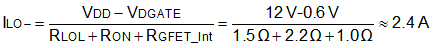SLUSCE9B June   2017  – March 2020

PRODUCTION DATA.

1. Features
2. Applications
3. Description
1.     Device Images
4. Revision History
5. Pin Configuration and Functions
6. Specifications
7. Detailed Description
1. 7.1 Overview
2. 7.2 Functional Block Diagram
3. 7.3 Feature Description
4. 7.4 Device Functional Modes
8. Application and Implementation
1. 8.1 Application Information
2. 8.2 Typical Application
9. Power Supply Recommendations
10. 10Layout
11. 11Device and Documentation Support
12. 12Mechanical, Packaging, and Orderable Information

• D|8

8.2.2.5 Selecting Gate Resistor RON/ROFF

Resistor RON and ROFF are sized to achieve the following:

• Limit ringing caused by parasitic inductances and capacitances.
• Limit ringing caused by high voltage/current switching dV/dt, dI/dt, and body diode reverse recovery.
• Fine-tune gate drive strength to optimize switching loss.
• Reduce electromagnetic interference (EMI).

As mentioned in Output Stage, the UCC27712 has a pull up structure with a P-channel MOSFET providing a peak source current of 1.8A.

For this example 3.3-Ω resistors for RON and 2.2-Ω resistors for ROFF were selected to provide damping for ringing and ample gate drive current.

Equation 6.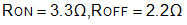Therefore the peak source current can be predicted with:

Equation 7.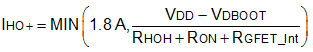Equation 8.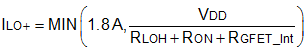where

• RON: External turn-on resistance
• RGFET_Int: Power transistor internal gate resistance, found in the power transistor datasheet.
• IO+ = Peak source current. The maximum values between 1.8 A, the UCC27712 peak source current, and the calculated value based on the gate drive loop resistance.

In this example:

Equation 9.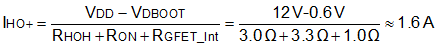Equation 10.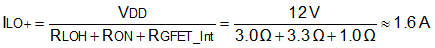Therefore, the high-side and low side peak source current is 1.6 A. Similarly, the peak sink current can be calculated with:

Equation 11.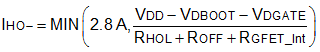Equation 12.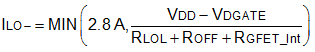where

• ROFF: External turn-off resistance
• VDGATE: The diode forward voltage drop which is in series with ROFF. The diode in this example is an MBRM130L.
• IO- = Peak sink current. The maximum values between 2.8 A, the UCC27712 peak sink current, and the calculated value based on the gate drive loop resistance.

In this example:

Equation 13.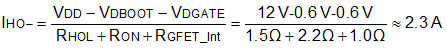Equation 14.